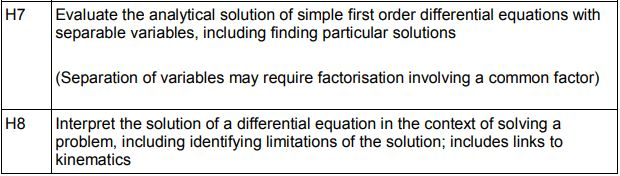# Differential EquationsThe study of Differential Equations is part of the prescribed core content of A Level further mathematics; this prescribed core comprising approximately 50% of each examination board’s content.

There are some very useful resources on this subject, particularly needed for further mathematics, where for many teachers they will be teaching content new on the specification.Teaching the MEI OCR specification, I do find the Delivery Guides and Check in tests useful, these provide both teaching guidance and example questions.On Dr Frost’s excellent site you will find resources on Differential Equations, see Chapter 11, Integration for Mathematics and Chapters 7 and 8 on Further Mathematics.

We can use a little technology to check solutions to Differential Equations using WolframAlpha. The slides here illustrate the syntax for first and second order differential equations. Examples like this and more are available from WolframAlpha: Examples for Differential Equations.

Differential Equations on Slideshare.

On the subject of Technology, we can have a look at Edexcel’s helpful guide with links to numerous GeoGebra files: A Guide to using GeoGebra when teaching AS and A Level Mathematics.which includes Solving Differential Equations.

Notes and examples on Differential Equations.